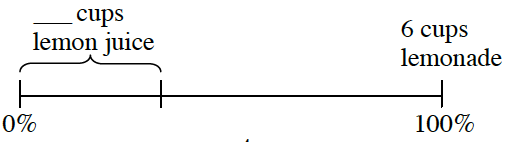### Home > CC2 > Chapter 5 > Lesson 5.2.4 > Problem5-62

5-62.

A lemonade recipe calls for using a ratio of $2$ cups of lemon juice for every $4$ cups of water.1. Draw and label a diagram like the one above to show the percent of lemonade that is water and the percent that is lemon juice.

Based on the ratio, how much lemon juice and water would be needed to make $6$ cups of lemonade?

2. What is the ratio of lemon juice to total liquid?

How much lemon juice is required with every $4$ cups of water to create $6$ cups of lemonade?

3. Angel made $10$ cups of lemonade. She used $3$ cups of lemon juice in her mixture. Did she follow the same recipe? In other words, did she use the same ratio of lemon juice to total liquid?

Is $3$ cups of lemon juice for $10$ cups of lemonade the same ratio that you found above? Test to see if Angel followed the same mixture ratio by checking if his ratio is equivalent to the recipe given.

She did not; $\frac{3}{10}\ne\frac{2}{6}$ because the two ratios cannot be related by a Giant One.